# Model PLLs in the Phase Domain

This example shows how to model a phase-locked loop (PLL) in the phase domain, compare the analytic results to simulation results in the time domain, and identify the advantages and disadvantages of each approach. Most PLL analysis is performed in the phase domain, and this example shows you how to produce a complete analysis using a minimum of time and effort.

The phase domain analysis calculates the PLL transfer function, loop error function, lock time and noise transfer impedances using the Control System Toolbox™. (Automated loop tuning is described in the Tune Phase-Locked Loop Using Loop-Shaping Design example.)

The PLL design and the time domain simulation model were derived from the Phase Noise at PLL Output example.

The general loop filter modeling procedure uses the Mixed-Signal Blockset™ Linear Circuit Wizard and was derived from the Circuit Design Details Affect PLL Performance example.

### Phase Domain Block Models

This section shows how to create the phase domain models for the individual blocks of an analog phase/frequency locked loop.

The term phase domain refers to the representation of a periodic signal in terms of its phase with respect to an ideal reference with the same period, instead of in terms of its voltage or current as a function of time. Most PLL analyses (in contrast with simulations) treat the PLL as a linear control system in the frequency domain, with the reference signal and the output of the VCO/divider represented in the phase domain.

The Control System Toolbox supports both transfer function (tf) and state space (ss) models. While the tf objects tend to be easier to use, the ss objects are often more accurate at higher frequencies.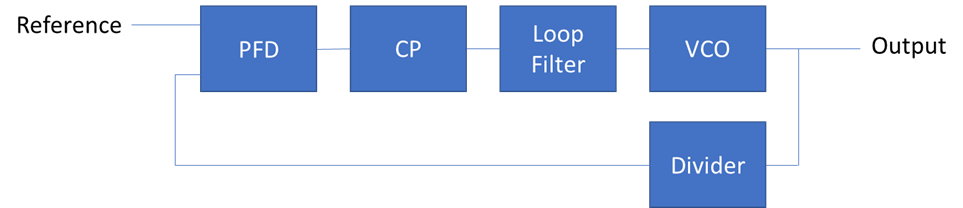To enable comparison of analysis results to time domain simulation results, this example uses the same design values as the Phase noise at PLL output example.

PllKphi = 5e-3; % Charge Pump output current PllKvco = 100e6; % VCO sensitivity PllN = 70; % Prescaler ratio PllR2 = 1.33e3; % Loop filter resistance for second order response (ohms) PllR3 = 1.7e4; % Loop filter resistance for third order response (ohms) PllR4 = 0; % Loop filter resistance for fourth order response (ohms) PllC1 = 1.31e-11; % Loop filter direct capacitance (F) PllC2 = 1.44e-10; % Loop filter capacitance for second order response (F) PllC3 = 9.41e-13; % Loop filter capacitance for third order response (F) PllC4 = 0; % Loop filter capacitance for fourth order response (F) 

Reference

The reference signal is assumed to have the same frequency as the ideal reference used to define the phase domain. However, there can be a time-varying phase offset between the reference signal and the ideal reference. This phase offset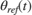, orin the Laplace domain, is an input to the linear control system.

VCO and Clock Divider

The VCO output phase is the integral of the VCO control voltage.Or, in the Laplace domain,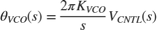From this equation, the statement to construct an ss model of the VCO is

vco = ss(0,2*pi*PllKvco,1,0); 

Given a divider ratio PllN (not necessarily integer), the ss object for the divider is

divN = ss(0,0,0,1/PllN); 

The VCO also has phase noise at its output, characterized as a phase noise density, filtered by the closed loop gain of the PLL.

Phase/Frequency Detector (PFD) and Charge Pump (CP)

Given phase domain inputsand, and maximum output current, the average output current of the PFD/CP is:Or in the Laplace domain:The CP also has phase noise at its output, characterized as a phase noise density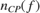.

Loop Filter

The loop filter presents a transfer impedanceto the CP output, thus converting the CP output current to a control voltage applied to the VCO. [ 1 ] describes a family of widely used second, third and fourth order loop filters. More convenient modeling of this and other loop filter circuit designs using the Linear Circuit Wizard is described in the General Loop Filters section below.

The second order filter is described by the equationThe higher order filters are formed by adding a series R/shunt C section. Given an k-1 order filter design for which Z_(k-1) (s)= (b(s))/(a_(k-1) (s)) , followed by series resistor R_k and shunt capacitor C_k, the transfer impedance of the higher order filter isOne way to transform these equations into an ss object is to calculate the transfer function coefficients and then use the tf2ss function. Given that the filter has nsections sections, with corresponding element values, the procedure is

nsections = 3; b = zeros(1,nsections+1); a = [PllR2*PllC1*PllC2, PllC1+PllC2, 0]; b(end-1:end) = [PllR2*PllC2, 1]; if nsections > 2 tmp = conv(b, [PllC3 0]); a = conv(a, [PllR3*PllC3, 1]) + tmp(end-3:end); end if nsections > 3 tmp = conv(b, [PllC4 0]); a = conv(a, [PllR4*PllC4, 1]) + tmp(end-4:end); end [A,B,C,D] = tf2ss(b,a); zlf = ss(A,B,C,D); 

The resistors in the loop filter also produce Johnson noise. This noise can be modeled as a noise current that is then applied to a transfer impedance to produce a noise voltage at the output of the loop filter. Given a resistance, the current spectral density is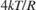Watts/Hz, where k is Boltzmann's constant and T is the absolute temperature.

The noise transfer impedance calculation follows a very similar process as that for the overall loop filter transfer impedance. However it must be calculated separately for each resistor in the loop filter. The function getNoiseTransferImpedance(R,C,n) included with this example produces an ss model for the device noise transfer impedance of the resistor.

### Control Loop Analysis

This section shows how to perform numerous open and closed loop analyses using the phase domain models of the PLL blocks. The process is to first create a closed loop system model and then invoke functions that perform the desired analysis using that model.

Most of the analyses only require a single input, single output model of the PLL, and so you will define that model first. The noise filtering analysis requires a multiple input model, and you will define that in the noise filtering section.

In PLL control loop modeling the divider ratio N is usually kept separate from the gain G due to the PFD/CP, loop filter, and VCO.The loop transfer function (from reference input to VCO output) is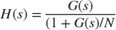The corresponding MATLAB code is

G = PllKphi/2/pi*zlf*vco; H = feedback(G,divN); H.InputName = 'ref'; H.OutputName = 'out'; 

Closed Loop Transfer Function

Use the bode function to plot the magnitude and phase of the closed loop transfer function.

NOTE: Configure the bode function to scale the frequency in Hz and not rad/s.

figure(1); opts = bodeoptions('cstprefs'); opts.FreqUnits = 'Hz'; bodeplot(H,opts); title('Closed Loop');You can also use other variants of the bode function to output results to the workspace for use in other analyses.

Loop Stability

Use the bode function again to plot the magnitude and phase of the loop gain, only this time use the series function instead of the feedback function

GoverN = series(G,divN); figure(2); bodeplot(GoverN,opts); title('Open Loop');Lock Time

Obtain the loop lock time from the loop step response.

figure(3); [ystep,tstep] = step(H); plot(tstep,ystep); title('Lock Time');Noise Filtering

The analysis of noise filtering usually requires multiple inputs- one for each noise source. While such a model could be used for all the analyses presented earlier in this section, that would complicate the coding without providing additional insight. The required block diagram is shown below.The procedure is to use the connect function to obtain a transfer function from each noise source to the PLL output, apply each of these transfer functions to their respective noise source, and then plot the resulting output noise spectral densities.

The zlf, vco, and divN blocks are already defined.

Define blocks required for noise filtering analysis.

R = [PllR2, PllR3, PllR4]; C = [PllC1, PllC2, PllC3, PllC4]; zr2 = getNoiseTransferImpedance(R,C,2); zr3 = getNoiseTransferImpedance(R,C,3); 

The example loop filter is third order. No need for zr4.

icp = ss(0,0,0, PllKphi/2/pi); sum1 = sumblk('ph = ref - fbk'); sum2 = sumblk('lfi = cp + nCP'); sum3 = sumblk('vcntl = lfo + nz2 + nz3'); sum4 = sumblk('out = vo + nVCO'); % zlf.InputName = 'lfi'; zlf.OutputName = 'lfo'; vco.InputName = 'vcntl'; vco.OutputName = 'vo'; divN.InputName = 'out'; divN.OutputName = 'fbk'; icp.InputName = 'ph'; icp.OutputName = 'cp'; zr2.InputName = 'nR2'; zr2.OutputName = 'nz2'; zr3.InputName = 'nR3'; zr3.OutputName = 'nz3'; 

Connect the PLL as a control system.

Hnoise = connect(icp,zlf,vco,divN,zr2,zr3,sum1,sum2,sum3,sum4,... {'ref','nCP','nR2','nR3','nVCO'},'out'); Href = Hnoise(1,1); Hncp = Hnoise(1,2); Hnr2 = Hnoise(1,3); Hnr3 = Hnoise(1,4); Hnvco = Hnoise(1,5); 

Plot the transfer functions

figure(4); opts.PhaseVisible = 'off'; bodeplot(Href,Hncp,Hnr2,Hnr3,Hnvco,opts); title('Pll Noise Transfer Functions'); legend('ref to out','CP to out','R2 to out','R3 to out','VCO to out');Output Noise Spectral Density

Each of the PLL's internal noise sources can have a flicker noise component (1/f) as well as an additive white Gaussian noise (AWGN) component that is flat with frequency. In this section you will model the combination of these two components as an AWGN process driving a single filter with a gain that is unity at high frequencies but increases as 1/f below a predefined corner frequency. The getFlickerFilter function supplied with this example provides a useful approximation.

The model for the VCO phase noise n_VCO can be obtained from the measured or specified phase noise of the VCO using the process defined for the MSB Ring Oscillator model. This process fits the measured data to a physical model of the VCO (including flicker noise) while avoiding a couple of inaccuracies present in most oscillator phase noise measurements. While the Ring Oscillator model process produces a period offset standard deviation, the application to phase domain modeling requires that the period offset be scaled to a phase offset.

You can derive the n_VCO model for the time domain simulation used in this example using the following steps.

PllFoffset = [30e3 100e3 1e6 3e6 10e6]; % VCO offset frequencies (Hz) PllPhaseNoise = [-56 -106 -132 -143 -152]; % VCO output phase noise (dBc/Hz) CfgRef = 30e6; % Reference frequency f0 = PllN*CfgRef; [periodjitter,fcorner] = ... msblks.VCO.estimatePhaseNoiseCore(f0,PllFoffset,PllPhaseNoise); phasejitter = 2*pi*f0*periodjitter; % Convert from period jitter to phase jitter % Scale for the fact that the phase jitter occurs in one VCO output cycle. n_VCO = ss(0,sqrt(f0)*phasejitter,1,0) * getFlickerFilter(fcorner); 

You can model the Johnson current noise in the loop filter resistors by multiplying the broadband current noise density times a flicker filter. Due to the high quality of modern manufacturing, the typical corner frequency for a resistor is somewhere between 1kHz and 10kHz.

kB = 1.3805e-23; % Boltzmann's constant temp = 273 + 25; % Temperature, in degrees Kelvin for an ambient of 25C fc_resistors = 3e3; % Typical resistor flicker corner frequency n_r2 = sqrt(4*kB*temp/PllR2)*getFlickerFilter(fc_resistors); n_r3 = sqrt(4*kB*temp/PllR3)*getFlickerFilter(fc_resistors); 

The CP is an active circuit, and the noise it generates is determined by details of the circuit design and device parameters. Its noise density is best obtained from device data sheets. However at least one version of such data data sheet parameters [ 1 ] characterizes the noise at the output of the PLL and not the output of the charge pump.

For the sake of having some sort of model, suppose that

1. In steady state operation, on average one or the other CP output transistor is operating with a duty cycle d. 0.01 < d < 0.1

2. When one of the CP output transistors is on, itschannel conductivityiswhere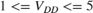and the saturation factor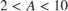.

3. The channel current integration constant isThen the CP current noise spectral density isCP_d = 0.03; CP_A = 4; CP_VDD = 5; CP_gamma = 1; n_CP = sqrt(4*kB*temp*CP_d*CP_gamma*PllKphi/CP_A/CP_VDD)*... getFlickerFilter(fc_resistors); 

Plot the phase noise contributions for all four noise sources.

figure(5); opts.PhaseVisible = 'off'; opts.MagLowerLimMode = 'manual'; opts.MagLowerLim = -200; opts.XLimMode = 'manual'; opts.XLim = [100,1e9]; nout_CP = n_CP*Hncp; nout_R2 = n_r2*Hnr2; nout_R3 = n_r3*Hnr3; nout_VCO = n_VCO*Hnvco; bodeplot(nout_CP,nout_R2,nout_R3,nout_VCO,opts); title('Pll Phase Noise'); legend('CP to out','R2 to out','R3 to out','VCO to out'); opts.PhaseVisible = 'on'; opts.MagLowerLimMode = 'auto'; opts.XLimMode = 'auto';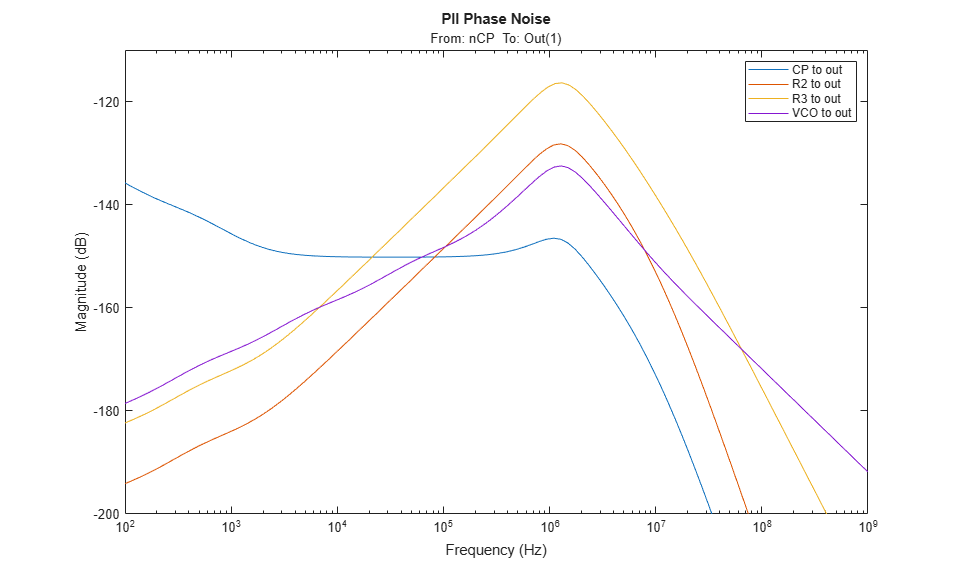### Compare to time domain results

This section compares the phase domain analysis results to the results of a time domain simulation using the model presented in the Phase Noise at PLL Output example. The clearest and most direct approach is to compare the step response predicted by the analysis with the phase lock response produced by the simulation.

The PllPhaseNoiseExample.slx model included with this example is statically configured for the purposes of this section. The workspace output and a CP output monitor port give you access to additional data when performing follow-on investigations such as are suggested in this section and the Compare Z domain with Laplace domain subsection.

Run the time domain simulation and compare the loop lock response to a manually adjusted copy of the phase domain step response.

open_system('PllLoopDynamicsExample.slx'); out = sim('PllLoopDynamicsExample.slx'); figure(6); plot(out.vco_in.Time,squeeze(out.vco_in.Data)); hold on; plot(tstep+1.46e-7,0.913/24.7*(ystep-70)); title('Comparison between Simulation and Analysis') legend('Time domain simulation','Phase domain analysis')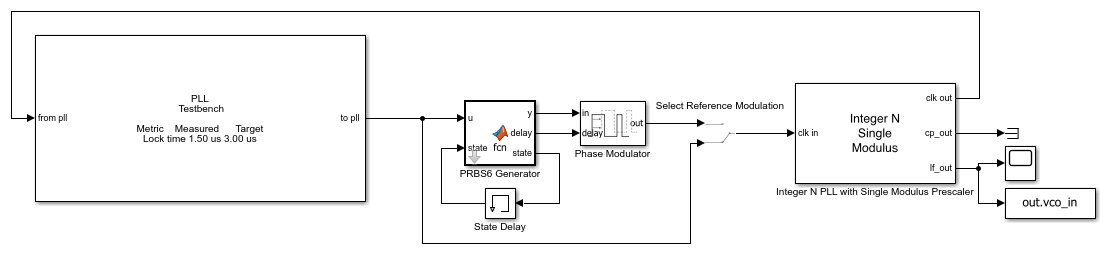The time domain loop lock response agrees quite well with the phase domain analysis result. It is interesting that there is a portion of the time domain response that precedes the phase domain step response. Using features explained in the following paragraph, you can demonstrate that this phenomenon occurs even for very small phase steps, and is therefore a linear response phenomenon.

As an aid to further study, the reference phase modulator in the model has been configured to produce a PRBS6 modulation with a long enough period to capture the entire step response, and a low enough amplitude to assure operation within the linear range. You can explore the resulting behaviors by toggling the switch at the output of the reference phase modulator, and you may also find it helpful to examine the CP output to verify operation in the linear range.

Because of the very high loop bandwidth, some leakage of the high frequency portions of the CP output are clearly visible in the loop filter output. These high frequency perturbations modulate the VCO frequency and cause spurious responses at the output of the PLL. Phase domain analysis does not address these spurious responses.

### General Loop Filters

This section demonstrates how to use the Linear Circuit Wizard to obtain ss objects for detailed loop filter designs, including their device noise transfer impedances.

The Linear Circuit Wizard reads a SPICE netlist and then sets up and solves Kirchhoff%s equations algorithmically. Several different outputs are available including a report of poles, zeros, and gains. You will transform these poles, zeros, and gains into an ss object.

While this example supplies a SPICE netlist for the one loop filter that is used throughout the example, you can apply the following procedure to the SPICE netlist for any of a wide variety of circuits.

1. Open the model PllLoopDynamicsLCW.slx.

open_system('PllLoopDynamicsLCW.slx');2. Open the mask for the Linear Circuit Wizard.

3. Select the Browse function and then select the SPICE file LoopFilter.sp.

4. Select the Parse netlist and redefine ports function.

5. Go to the Port Definition tab and make sure that the desired input and output ports were configured by the netlist. If they were not configured by the netlist, you can use the Ports tab to add, delete, or modify ports.

6. Go to the Device noise tab and enable the device noise for any potential device noise sources you want to have modeled. In this example, enable the device noise generator for the resistors R2 and R3.

7. Select the Output poles and zeros function. A structure LinearCircuitWizardPoleZero is added to your workspace.

For use in the following transformations, a copy of LinearCircuitWizardPoleZero has also been saved to the file PllLCWPoleZero.mat included with this example.

The following transforms a set of poles, zeros and gains into a separate zpk model and then ss model for each transfer impedance. Note that the Gains are low frequency gains, not including poles or zeros at the origin, while the k factor is based on a different formulation of the transfer function. The gain2k function is supplied with this example to aid you in performing the gain to k transformation.

load('PllLCWPoleZero.mat'); p = LinearCircuitWizardPoleZero.Poles; z = LinearCircuitWizardPoleZero.Zeros; g = LinearCircuitWizardPoleZero.Gains; k = gain2k(p,z,g); [A,B,C,D] = zp2ss(z{1},p,k(1)); % Loop filter transfer impedance zlf_lcw = ss(A,B,C,D); [A,B,C,D] = zp2ss(z{2},p,k(2)); % R2 transfer impedance zr2_lcw = ss(A,B,C,D); [A,B,C,D] = zp2ss(z{3},p,k(3)); % R3 transfer impedance zr3_lcw = ss(A,B,C,D); 

Use bode plots to demonstrate that the transfer impedance models produced through the Linear Circuit Wizard are identical to those produced through direct symbolic expressions.

figure(7) bodeplot(zlf,zlf_lcw,zr2,zr2_lcw,zr3,zr3_lcw,opts); title('Comparison of manual models to Linear Circuit Wizard models');### Z Domain Modeling

This section presents modeling in the uniform sampled time domain (Z domain) as an alternative to modeling in the Laplace domain.

The PFD/CP only samples the phase difference between reference and output once every reference cycle [ 2, 3 ]. Thus the dynamics of the PLL are inherently tied to that uniform sample rate, and the mathematics that rigorously applies to a linear control loop analysis is Z domain mathematics. There are important behaviors such as spurious responses that occur at higher frequencies. However linear mathematics does not apply to those behaviors.

Given a Z domain expression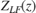of the loop filter response, the Z domain expression for the loop gain [ 3 ] isThe remaining task is to convert thefunction from the Laplace domain analysis to the Z domain. There are many ways to perform this task, and several of them have been implemented in the MATLAB c2d function. The appropriate option in this application is zero-order hold, the default for the c2d function. Note that the Z domain modeling does require an explicit sample time value equal to the period of the reference signal.

fref = 30e6; ts = 1/fref; vcoz = ss(1,ts*PllKphi*PllKvco,1,0,ts); divNz = ss(0,0,0,1/PllN,ts); zlfz = c2d(zlf,ts); Gz = vcoz*zlfz; Hz = feedback(Gz,divNz); Hz.InputName = 'ref'; Hz.OutputName = 'out'; GzoverN = Gz*divNz; 

Compare Z domain with Laplace domain

It is informative to compare the Z domain predictions of the closed loop, open loop, and step responses with the corresponding results from the Laplace domain analysis.

figure(1); bodeplot(H,Hz,opts); title('Closed Loop'); legend('Laplace domain','Z domain'); figure(2); bodeplot(GoverN,GzoverN,opts); title('Open Loop'); legend('Laplace domain','Z domain'); figure(3); step(H,Hz); title('Lock Time'); legend('Laplace domain','Z domain');In this comparison, note that while the frequency response and step response from the Z domain analysis are very similar to those obtained from the Laplace domain analysis, the phase margin predicted by the Z domain analysis is far less than the design value chosen as part of the design in the Laplace domain. This is a critical observation, as the loop phase margin is a fundamental requirement of the control loop design.

As stated in [ 2 ], this is a typical result for PLLs whose loop bandwidth equals or exceeds one tenth of the reference frequency. For much smaller loop bandwidths the difference between Z domain and Laplace domain is much smaller.

Note, however, that it is the Laplace domain analysis result that closely matches the time domain simulation. You might find this to be a suitable topic for further study.

+ Both open and closed loop linear control analyses are easy, fast, reliable and accurate.

+ You can readily include detailed phase noise modeling in your analysis, with the effect of each phase noise source indicated clearly and accurately.

+ Using the Linear Circuit Wizard, you can easily and accurately include detailed linear circuit designs, especially for the loop filter.

- Phase domain modeling is limited to one sample per reference cycle, and therefore a Nyquist frequency limit of one half the reference frequency. For example, it is not applicable to the analysis of spurious output responses past this Nyquist limit.

- While phase domain modeling can be applied to nonlinear behaviors such as frequency acquisition, such applications lose the inherent advantages of linear control analysis associated with phase domain modeling.

- Phase domain modeling is usually not applicable to models that combine the PLL with other circuits that use the output of the PLL.

### References

1. Dean Banerjee, PLL Performance, Simulation and Design, 5th edition, Texas Instruments, SNAA106C, May 2017.

2. P. K. Hanumolu, M. Brownlee, K. Mayaram and Un-Ku Moon, "Analysis of charge-pump phase-locked loops," in IEEE Transactions on Circuits and Systems I: Regular Papers, vol. 51, no. 9, pp. 1665-1674, Sept. 2004, doi: 10.1109/TCSI.2004.834516.

3. Mike Steinberger, "Discrete Time Modeling of PLLs", MathWorks document, May 31, 2022.